TUTORIALS
TUTORIALS HOME

GENERAL MATH
NOTATION & METHODS OF PROOF
INDUCTION
COMPLEX NUMBERS
POLYNOMIALS

LINEAR ALGEBRA
VECTORS
SYSTEM OF LINEAR EQUATIONS
MATRICES
EIGENVALUES & EIGENVECTORS
ORTHOGONALITY
VECTOR SPACE
DISTANCE & APPROXIMATION

HOME
TESTS
TUTORIALS
SAMPLE PROBLEMS
COMMON MISTAKES
STUDY TIPS
GLOSSARY
APPLICATIONS
MATH HUMOUR

### VECTOR SPACE TUTORIAL

This tutorial includes many theorems that involve vector spaces and other topics that apply to vector spaces. To gain the best understanding of the material covered it is suggested that you review each proof or if there is none, try to prove each theorem on your own. Also each topic has several examples that pertain to the theorems or definitions given and should also be reviewed.

# Vector Spaces

If we have a set V and u and v exist in V then V is said to be closed under addition if u + v exists in V

If v is in V, and k is any scalar, then V is said to be closed under scalar multiplication if kv exists in V

A vector space or linear space V, is a set which satisfies the following for all u, v and w in V and scalars c and d: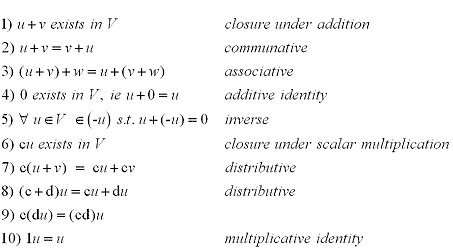Probably the most improtant example of a vector space is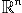for any n1. We can easily see that the additive identity 0 exists and it is closed under addition and scalar multiplication. To show thatsatisfies the other 8 properties very simple and is left as an exercise.

The examples given at the end of the vector space section examine some vector spaces more closely. To have a better understanding of a vector space be sure to look at each example listed.

Theorem 1: Let V be a vector space, u a vector in V and c a scalar then:
1) 0u = 0
2) c0 = 0
3) (-1)u = -u
4) If cu = 0, then c = 0 or u = 0

# Examples:

1 | Show if a set is a vector space
2 | 3 Examples of a vector spaces

# Subspaces

A subset W of a linear space V is called a subspace of V if:
1) W contains the additive identity 0
2) W is closed under addition
3) W is closed under scalar multiplication

Basically a subset W of a vector space V is a subspace if W itself is a vector space under the same scalars and addition and scalar multiplication as V.

Consider the set of vectors S={v1, v2, ... , vk} of a vector space V. Then we say the span of v1, v2, ... , vk is the set of all linear combinations of v1, v2, ... , vk and is denoted span(S). If V = span(S) then S is called a spanning set for V and V is spanned by S.

Theorem 2: Let v1, v2, ... , vk be vectors in a vector space V then:
1) span(v1, v2, ... , vk) is a subspace of V
2) span(v1, v2, ... , vk) is the smallest subspace of V that contains v1, v2, ... , vk

# Examples:

3 | Show that a set is a subspace

# Linear Independance

We have covered what linear independance is in previous tutorials but will now apply it to vector spaces.

We say a set of vectors v1, v2, ... , vk are linearly independent if the equation:

c1v1+ c2v2+...+ ckvk = 0 only has trivial solution: c1 = c2 = ... = ck = 0

If a set is not linear independent then it is said to be linearly dependent. This means that there is at least one ci in the above equation such that ci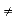0 where i = 1, 2, ... , k.

Theorem 3: A vector set {v1, v2, ... , vk} in a vector space V is linearly dependent if and only if at least one vi (where i = 1, 2, ... , k) can be written as the linear combination of the others.

We say that elements v1, v2, ... , vk form a basis of a vector space V if they span V and are linearly independent. This means that all v in V can be written uniquely as a linear combination ie:

v = c1v1+ c2v2+ ... + ckvk

The scalars or coefficients c1, c2, ... , ck are called the coordinates of v with respect to the basis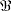= {v1, v2, ... , vk} and the column vector: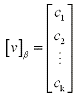is called the coordinate vector of v with respect toTheorem 4: Consider a basisfor a subspace V and let u , v be vectors in V and c a scalar. Then:
1) [u + v]B = [u]B + [v]B
2) [cu]B = c[u]B
4 | Proof

Theorem 5: Let V be a vector space with a basis= {v1, v2, ... , vk} and u1, u2, ... , uk be vectors in V. Then the set {u1, u2, ... , uk} is linearly independent in V if and only if
{[u1]B, [u2]B, ... , [uk]B} is linearly independent in.

# Examples:

4 | Find the basis

# Dimention

The dimention of a subspace V or dim(V) is the number of vectors in the basis of V. Finite-dimentional defines a basis which has a finite number of vectors and infinite-dimentional defines a basis which has infinite number.

Theorem 6: If a subspace V has a basis= {v1, v2, ... , vk} then:
1) Any set of more then k vectors in V must be linearly dependent
2) Any set of fewer then k vectors in V cannot span V
3) Every basis for V has exactly k vectors
6 | Proof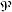is the set of all polynomials and has a basis: {1, x, x2, ... }. Since the basis has an infinte number of elements thenhas infinte dimention.n on the other hand contains n + 1 vectors in the basis, therefore is finite-dimentional

Theorem 7: If W is a subspace of a finite dimentional vector space V then:
1) W is finite dimentional and dim(W)dim(V)
2) dim(W) = dim(V) if and only if W = V

# Examples:

5 | Find a basis and determine the dimention

# Change of Basis

If we had two bases= {u1, u2, ... , un and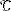= {v1, v2, ... ,vn} for a vector space V then there is a n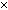n matrix whose columns are the coordinate vectors [u1]C, [u2]C, ... , [un]C of the vectors inwith respect tois called the change-of-basis matrix fromto. It is denoted by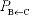That is:= [[u1]C, [u2]C, ... , [un]C]

This may seem complicated, but simplily put the columns ofare just the corrdinate vectors obtained by writing the "old basisin terms of the new basis. Try some of the examples in order to see how this is applied.

Theorem 8: If we had two basesandfor a vecor space V and change-of-basis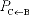then:
1)[x]B = [x]C for all x in V.
2)is the unique matrix P with the property that P[x]B = [x]C for all x in V.
3)is invertible and ()-1 =8 | Proof

Gauss-Jordan elimination is regularily used to find the inverse of a matrix. Finding the change-of-basis matrix from a standard basis requires the calculation of a matrix inverse. Therefore with a slight modification we can use the Gauss-Jordan method to find the change-of-basis matrix between two nonstandard bases. The following theorem explains how.

Theorem 9: Consider a subspace V with bases={u1, u2, ... , un} and={v1, v2, ... , vn}. If B = [[u1]E, [u2]E, ... , [un]E] and C = [[v1]E, [v2]E, ... , [vn]E] where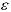is any basis for V then row reduction applied to [ C | B ] produces:

[ C | B ]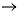[ I |]

# Examples:

6 | Find the change-of-basis matrix

# Linear Transformations

Consider two vector spaces V and W a function or mapping T from V to W is called a linear transformation if for all u, v in V and all scalars k:
1) T(u + v) = T(u) + T(v)
2) T(ku) = kT(u)

A mapping T:V to W is also a linear transformation if and only if for all v1, v2, ... ,vn in V and scalars c1, c2, ... , cn

T(c1 v1 + c2 v2 + ... + cn vn) = c1T( v1) + c2T( v2) + ... + cnT( vn)

Theorem 10: If T is a linear transformation from V to W and u and v exist in V then:
1) T(0) = 0
2) T(-v) = -T(v)
3) T(u - v) = T(u) - T(v)
10 | Proof

Theorem 11: Consider a linear transformation T from V to W letting= {v1, v2, ... , vn} be a spanning set for V. Then T() = {T(v1), T(v2), ... , T(vn)} spans the range of T.
11 | Proof

# Examples:

7 | Show whether a transformation is linear
8 | Show whether a transformation is linear

# Composition of a Linear Transformations

The compostion of a linear transformation is similar to the composition of a function in calculus.
If T is a linear transformation from U to V and S is a linear transformation from V to W then the composition of S with T is the transformation or mapping ST defined by

ST(u) = S(T(u) where u exists in U

The next theorem follows directly from the definition:

Theorem 12: If T from U to V and S from V to W are linear transformation then ST from U to W is a linear transformation.

A linear transformation T from V to W is invertible if there exists a linear transformation T' from W to V such that

T'T = I V and TT' = IW

Properties of inverses: If T is a linear transformation from V to U then:
1) If T is invertible then so is T'
2) If T is invertible then it's inverse is unique
3) T is invertible if and only if ker(T)={0} and im(T)=W

# Examples:

9 | Show whether a transformation is invertible

# Kernel and Range of a Linear Transformation

Consider a linear transformation T that goes from V to W. Then the kernel of T or the ker(T), is the set of all vectors which takes T to 0 or the null set in W. This can be shown in the following:

ker(T) = {v in V: T(v) = 0}

Another useful definition in this unit is the range of T, also refered to as the image of T and is denoted range(T). It is similar to the range of a function in calculus in that it is the set of all vectors in W which are images of the vectors in V under T. This can be shown in the following:

range(T) = {w in W: w = T(v for some v in W)

Theorem 13: The Rank-Nullity Theorem Consider the linear transformation T from V to W where V and W are finite dimentional then:

nullity(T) = dim(ker(T))    and    rank(T) = dim(range(T))
Then:
rank(T) + nullity(T) = dim(V)

13 | Proof

# Examples:

10 | Find the image and kernel of the transformation

# One-to-One and Onto Linear Transformations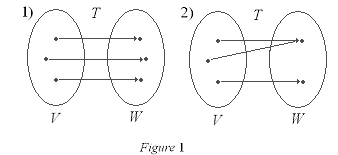Consider a linear transformation T from V to W. T is said to be one-to-one if for all u and v in V:

uvT(u)T(v)
and
T(u) = T(v)u = v

Figure 1 shows two examples of one-to-one functions. Both are also onto (see the next definition).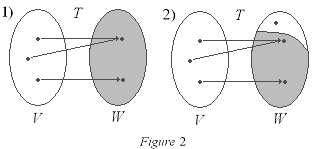A linear transformation T from V to W is said to be onto if for all w and W there is at least one v in V such that::

w = T(v)

Figure 2 has two examples. the first is onto beacause every point in W exists in V. The second example is not onto, beacause there is one point in W which does not corresponded to a point in V by T.

The following theorems can be used to solve various problems dealing with linear transformations. Before trying to manipulate the theorems it maybe better to view each proof first to gain a better understanding of each theorem.

Theorem 14: A linear transformation is one-to-one if and only if ker(T)={0}
14 | Proof

Theorem 15: Let dim(V) = dim(W) = n. Then a linear transformation T from VW is one-to-one if and only if is onto
15 | Proof

Theorem 16: Consider a one-to-one linear transformation T from VW. Then if we have a linearly independent set S={v1, v2, ... , vk} then T(S)={T(v1), T(v2), ... , T(vk)} is also linearly independent in W.
16 | Proof

Theorem 17: A linear transformation is invertible if and only if it is both one-to-one and onto
17 | Proof

# Examples:

11 | Find if the linear transformation is one-to-one and onto
12 | Show that the transformation is invertible

# Isomorphisms of Vector Spaces

An invertible linear transformation is called an isomorphism. We say that the linear spaces V and W are isomorphic if there is an isomorphism from V to W.

Properties of isomorphisms:
Consider a linear transformation T from V to W
1) If T is an isomorphism, the so is T-1
2) T is an isomorphism if and only if   ker(T) = {0} and range(T) = W
3) If v1, v2, ... , vk is a basis in V then T(v1), T(v2), ... , T(vk) is a basis in W
4) If V and W are finite dimentional vector spaces, then V is isomorphic to W if and only if
dim(V) = dim(W)

# Examples:

13 | Show that the transformation is an isomorphism

# Matrix of a Linear Transformation

Consider a linear transformation T fromtoand a basisof. The nn matrix B that transforms [x]B into [T(x)]B is called the-matrix of T for instance for all x in:

[T(x)]B = B[(x)]B

Constructing a-matrix B of a linear transformation T column by column is easy. If we had a vector x insuch that: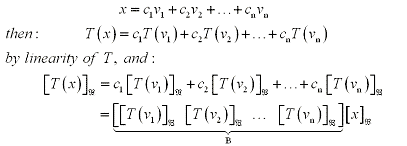Consider a linear transformation T fromtoand a basisofconsisting of vectors v1, v2, ... , vn. Then the columns of B are the-coordinate vectors of T(v1), T(v2), ... , T(vn). Then the-matrix of T is:

B=[[T(v1)]B, [T(v2)]B, ... , [T(vn)]B]

To clear things up a bit look at the following diagram. Say we had a basisof a subspace V of, consisting of vectors v1, v2, ... , vm. Then any vector x in V can be written uniquely as :

x = c1v1, c2v2, ... , cmvm

the scalars are called the-coordinates of x and the-coordinate vector of x denoted by [x]B is would be: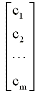Note that: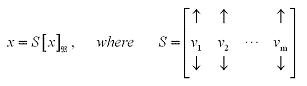Then our diagram looks like this: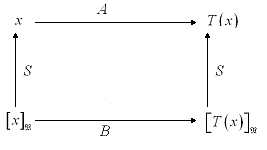where T(x) = A(S[x]B) and also T(x) = S(B[x]B) so that A(S[x]B) = S(B[x]B) for all x. Thus:

AS = SB, B = S-1AS and A = SBS-1

Consider two nn matrices A and B. We say that A is similar to B if there is an invertible matrix S such that:

AS = SB or B = S-1AS

Similarity relations:
1) An nn matirx A is simlar to itself (REFLEXIVE)
2) If A is similar to B then B is similar to A (SYMMETRY)
3) If A is similar to B and B is similar to C then A is similar to C (TRANSITIVITY)

Theorem 18: The Fundamental Theorem of Invertible Matrices:
Let T be a linear transformation from V to W and A be an nn matrix such that T(x) = Ax for any x in V. Then the following statements are equivalent: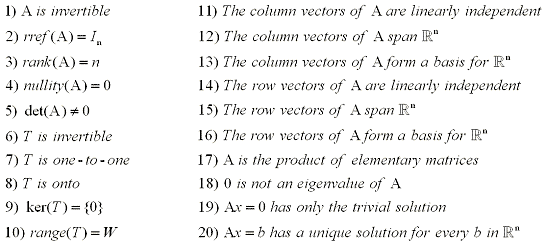# Examples:

14 | Find the matrix of the transformation
15 | Three part question

NOTE: This website has been designed for and tested for functionality on Internet Explorer 6.0, Netscape Navigator 7.2, Netscape Browser 8.0, and Mozilla Firefox 1.0. Other browsers may not display information correctly, although future versions of the abovementioned browsers should function properly.

COURSE HOMEPAGE
MATH 1046

FACULTY HOMEPAGES
Alex Karassev
Ted Chase